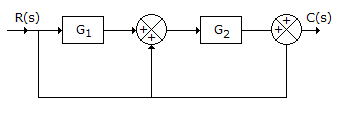# Electronics and Communication Engineering - Automatic Control Systems - Discussion

3.

For the system in the given figure. The transfer function C(s)/R(s) is[A]. G1 + G2 + 1 [B]. G1 G2 + 1 [C]. G1 G2 + G2 + 1 [D]. G1 G2 + G1 + 1

Explanation:

Transfer function = (G1 + 1) G2 + 1 = G1 G2 + G2 + 1.

 Beu said: (Apr 25, 2014) No. of fwd path = 3. No. of loop = 0.

 Rithika said: (Jun 27, 2014) Can anyone please solve it briefly ?

 Bitra Hanumantharao said: (Jul 9, 2014) Number of forward paths are 2. Number of loops are 2. That too no loop are not repeated. The transfer function of the system is: G1G2/ (1-G1G2-G2).

 Rajarajan said: (Oct 31, 2014) The above answer is not correct. Number of forward path = 2. Number of loops = 1. Answer is [G1G2+G2]/[1-G2].

 Roshan said: (May 5, 2015) There is only one loop. Number of +ve loop = 1. So, (G1G2 + G2) / (1 - G2).

 Anish Kumar said: (May 27, 2015) Answer (G2+G1G2)/(1-G2). Explanation: Forward path = 2 (G2 & G1G2). Loop = 1(G2). Using Masson formula: We get (G2+G1G2)/(1-G2);

 Anish said: (May 27, 2015) If anyone knows any answer which is different from my answer please explain it clearly so that everyone will understand.

 R C Pandey said: (Jun 9, 2015) @Anish. You are calculating TF between C and are. So there is only one forward path and that is G1G2.

 Praveen said: (Jan 10, 2016) Can anyone say the correct answer please as soon as possible?

 Fizza said: (Jan 10, 2016) What is the difference between transfer function and characteristic equation?

 Khetendra Vashishtha said: (Jun 10, 2016) Forward path = 2 (G2 & G1G2). Loop = 1(G2). Using Masons formula: then TF = (G2 + G1.G2)/(1 - G2).

 Arnab Bhattacharya said: (Jun 27, 2016) Using block diagram reduction technique, shift the summing point from the left of G2 to its right. It will place a block of TF G2. Now the lower paths are just summed up to produce (G2+1). The upper path is G1G2. Both the paths are in parallel. So, the ultimate TF is. C(s) / R(s) = G1G2 + G2 + 1. So, answer (C) is correct.

 Hamed said: (Jul 24, 2016) Not correct. The answer is [(G1 + 1) G2]/[1 - G2].

 Hamed said: (Aug 2, 2016) Hello, I'm very sorry to the last comment because after searching the answer is correct. C(s) / R(s) = G1G2 + G2 + 1.

 Anurag said: (Feb 13, 2017) Suppose the middle summing point is considered T(s). T(s) = R(s)G1+R(s). C(s) = C(s)T(s)+G2T(s). Now replace T(s) with R(s)G1+R(s). C(s) = C(s)R(s)G1+C(s)R(s)+G2R(s)G1+G2R(s). C(s)-C(s)R(s)G1-C(s)R(s) = (G2G1+G2)R(s). C(s)/R(s)-C(s)(G1+1) = G2+G1G2. C(s)/R(s) = C(s)(1+G1 G2+G1G2. Please correct me.

 Lordm said: (Sep 17, 2017) Answer is (G1G2+G2)/(1-G2). The summer could be split into two summers; the left side being an unity gain signal and signal with gain G2 being added. The right side is positive unity feedback loop. These are then in cascade.

 Ammar Khan said: (Jun 10, 2019) Use simple block reduction. The first block G1 and the parallel path below that add just after G1 add up to give you G1+1. Replace this portion with G1+1. Now you also see that there is another parallel path from before G1+1 that adds up after G2. This parallel path will again add up to the gain of the forward path i.e (G1+1)'- G2. So we get (G1+1)'- G2 + 1 = G1G2 + G2 + 1 which is the answer.

 Yogendra said: (Jul 6, 2019) No loop is there, 3 forward path is g2 and g1g2 and 1 only.